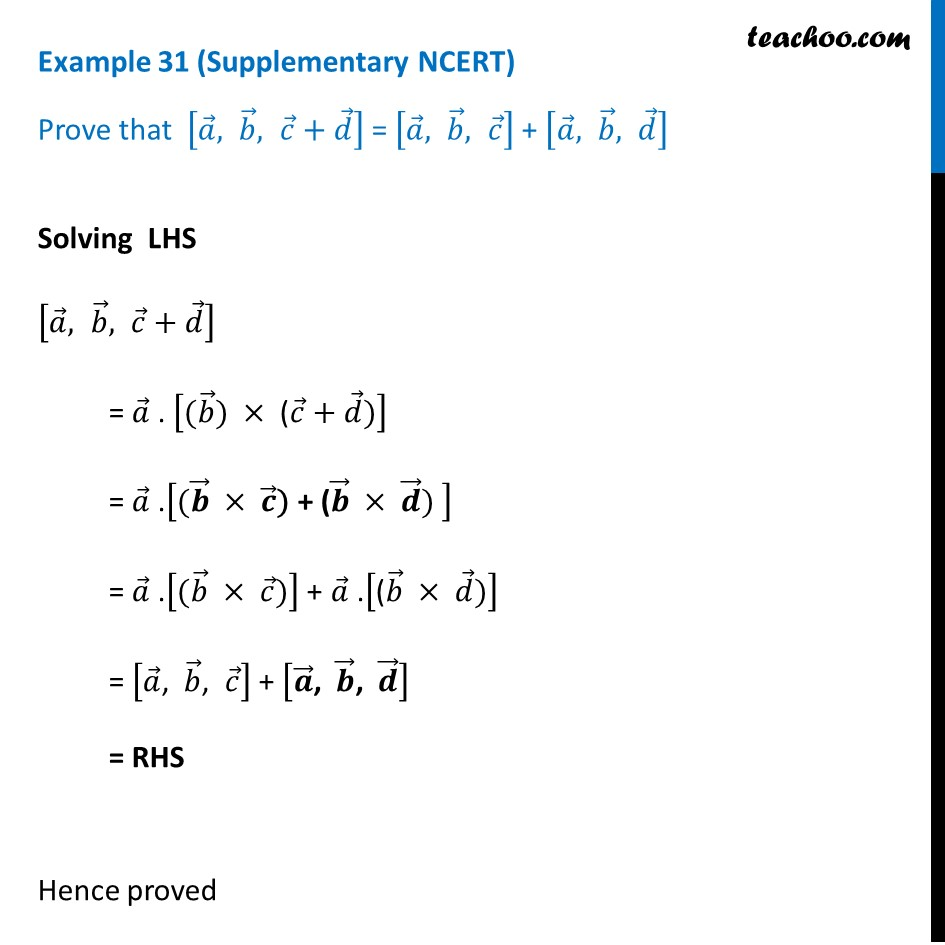1. Chapter 10 Class 12 Vector Algebra (Term 2)
2. Serial order wise
3. Examples

Transcript

Example 31 (Supplementary NCERT) Prove that [𝑎 ⃗, 𝑏 ⃗, 𝑐 ⃗+𝑑 ⃗ ] = [𝑎 ⃗, 𝑏 ⃗, 𝑐 ⃗ ] + [𝑎 ⃗, 𝑏 ⃗, 𝑑 ⃗ ]Solving LHS [𝑎 ⃗, 𝑏 ⃗, 𝑐 ⃗+𝑑 ⃗ ] = 𝑎 ⃗ . ["(" 𝑏 ⃗") " × "(" 𝑐 ⃗+𝑑 ⃗)] = 𝑎 ⃗ .["(" 𝒃 ⃗ × 𝒄 ⃗") + (" 𝒃 ⃗ × 𝒅 ⃗) ] = 𝑎 ⃗ .["(" 𝑏 ⃗ × 𝑐 ⃗")" ] + 𝑎 ⃗ .["(" 𝑏 ⃗ × 𝑑 ⃗)] = [𝑎 ⃗", " 𝑏 ⃗", " 𝑐 ⃗ ] + [𝒂 ⃗", " 𝒃 ⃗", " 𝒅 ⃗ ] = RHS Hence proved

Examples

Chapter 10 Class 12 Vector Algebra (Term 2)
Serial order wise